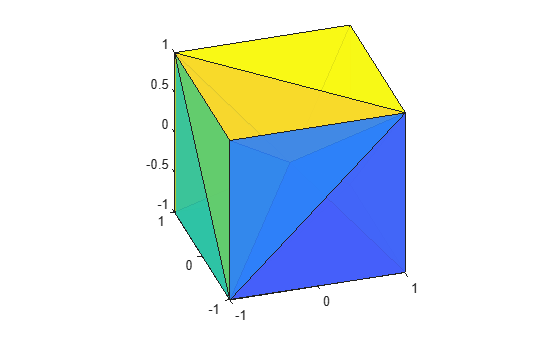# delaunayn

N-D Delaunay triangulation

## Syntax

```T = delaunayn(X) T = delaunayn(X,options) ```

## Description

`T = delaunayn(X)` computes a set of simplices such that no data points of `X` are contained in any circumspheres of the simplices. The set of simplices forms the Delaunay triangulation. `X` is an `m`-by-`n` array representing m points in n-dimensional space. `T` is a `numt`-by-(`n+1`) array where each row contains the indices into `X` of the vertices of the corresponding simplex.

`T = delaunayn(X,options)` specifies a cell array of options. The default options are:

• `{'Qt','Qbb','Qc'}` for 2- and 3-dimensional input

• `{'Qt','Qbb','Qc','Qx'}` for 4 and higher-dimensional input

If `options` is `[]`, the default options used. If `options` is `{''}`, no options are used, not even the default.

## Visualization

Plotting the output of `delaunayn` depends of the value of `n`:

## Examples

collapse all

This example generates an n-dimensional Delaunay triangulation, where `n = 3`.

```d = [-1 1]; [x,y,z] = meshgrid(d,d,d); % A cube x = [x(:);0]; y = [y(:);0]; z = [z(:);0]; % [x,y,z] are corners of a cube plus the center. X = [x(:) y(:) z(:)]; Tes = delaunayn(X)```
```Tes = 12×4 4 3 9 1 4 9 2 1 7 9 3 1 7 5 9 1 7 9 4 3 7 8 4 9 6 2 9 1 6 9 5 1 6 4 9 2 6 4 8 9 ⋮ ```

You can use `tetramesh` to visualize the tetrahedrons that form the corresponding simplex. `camorbit` rotates the camera position to provide a meaningful view of the figure.

```tetramesh(Tes,X); camorbit(20,0)```